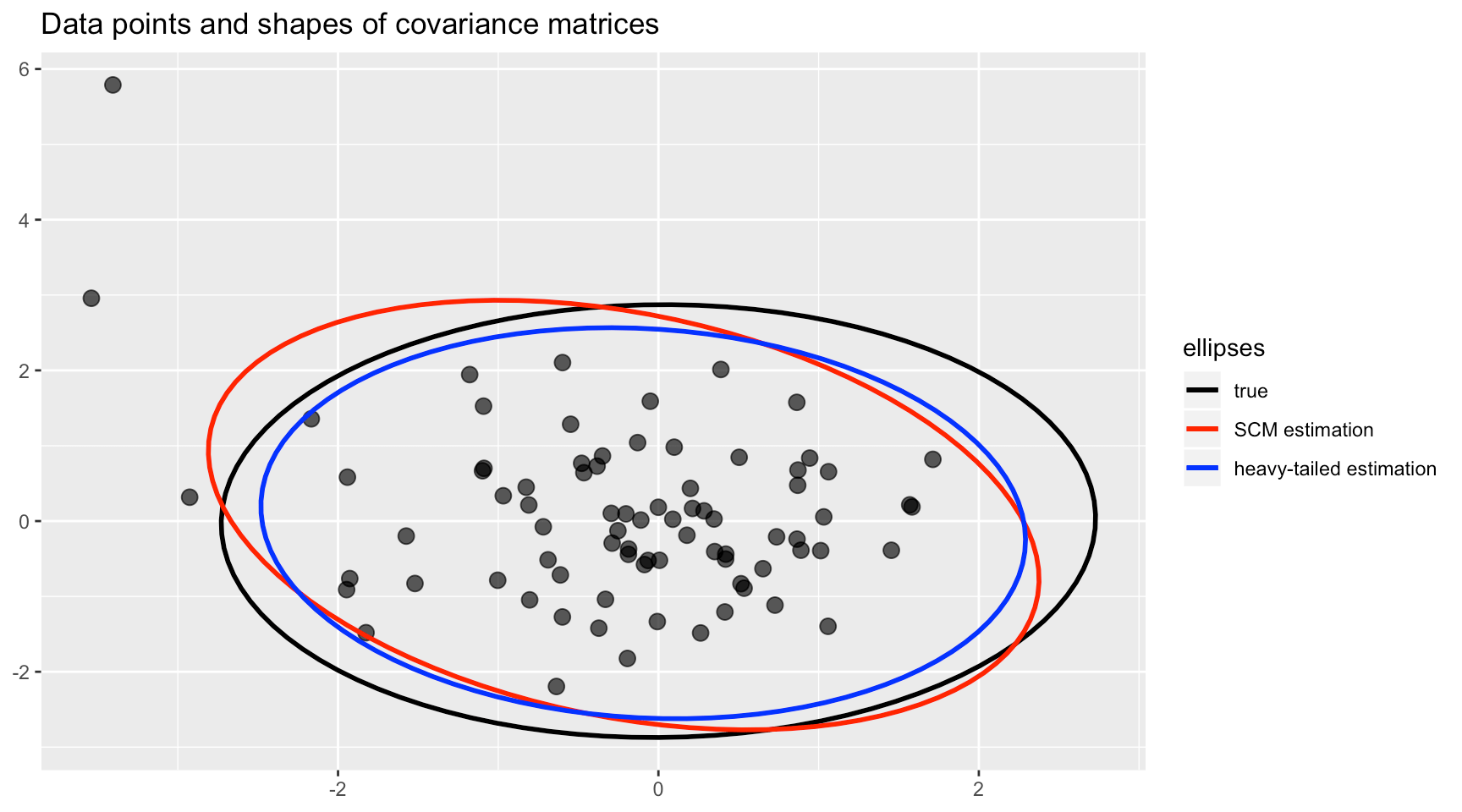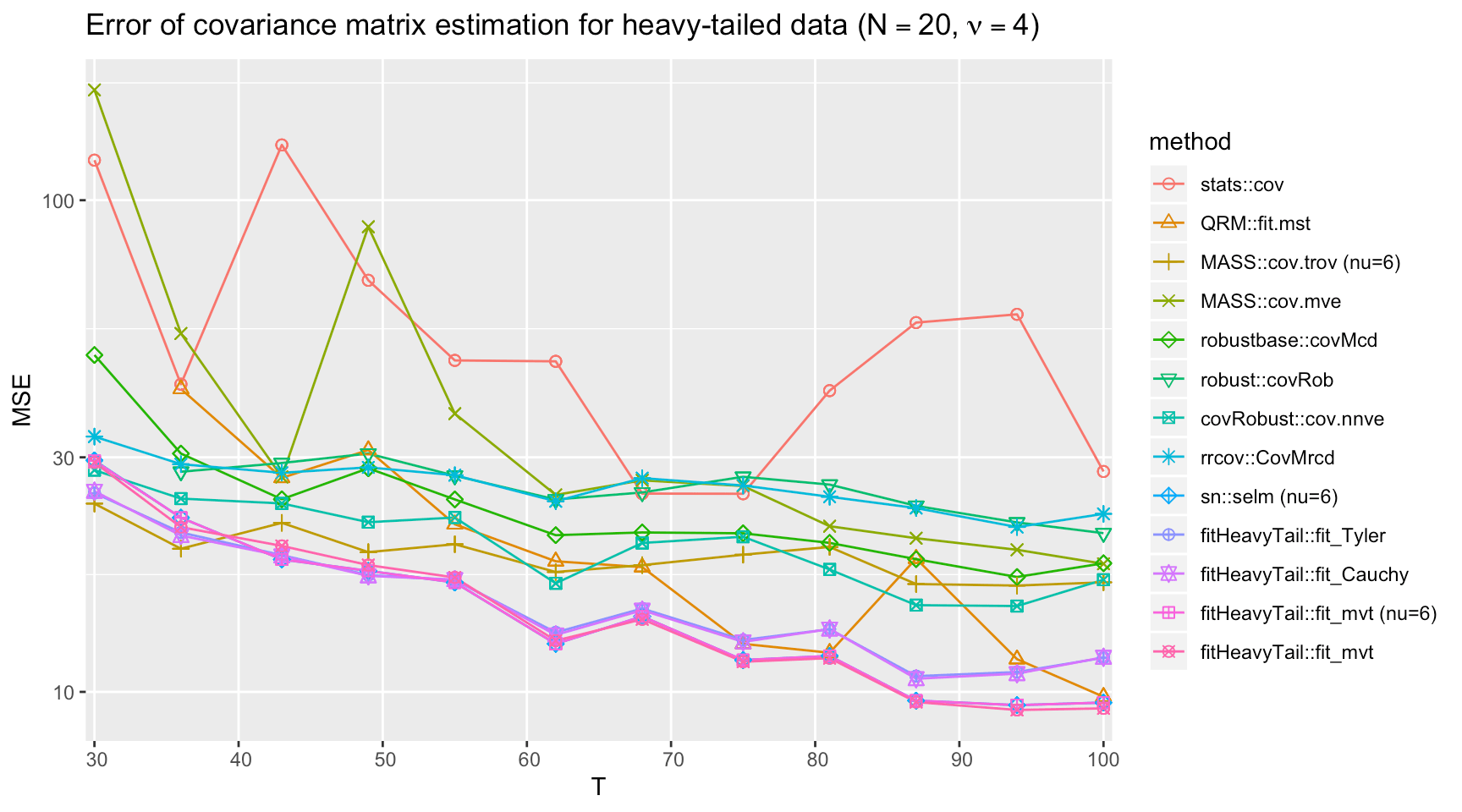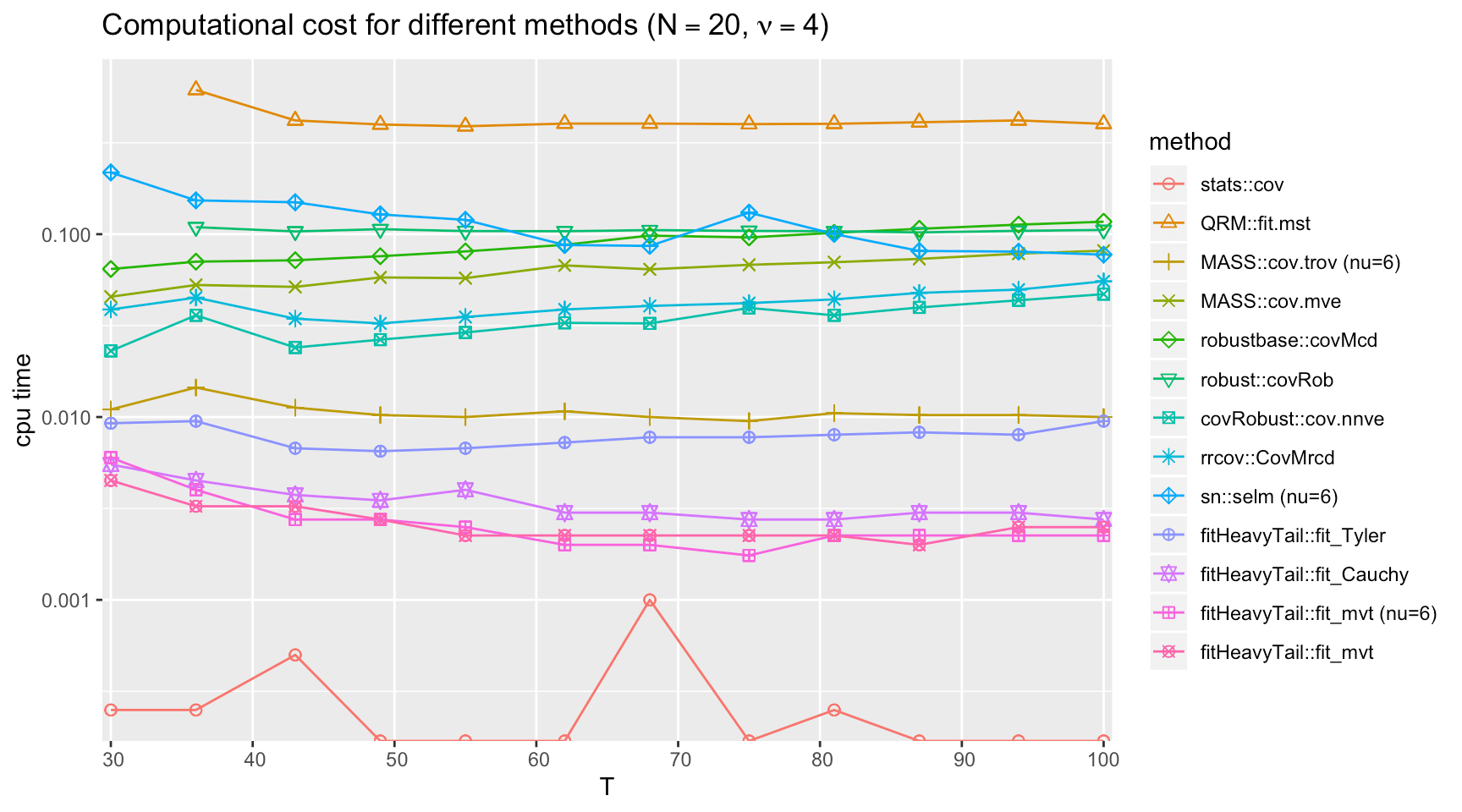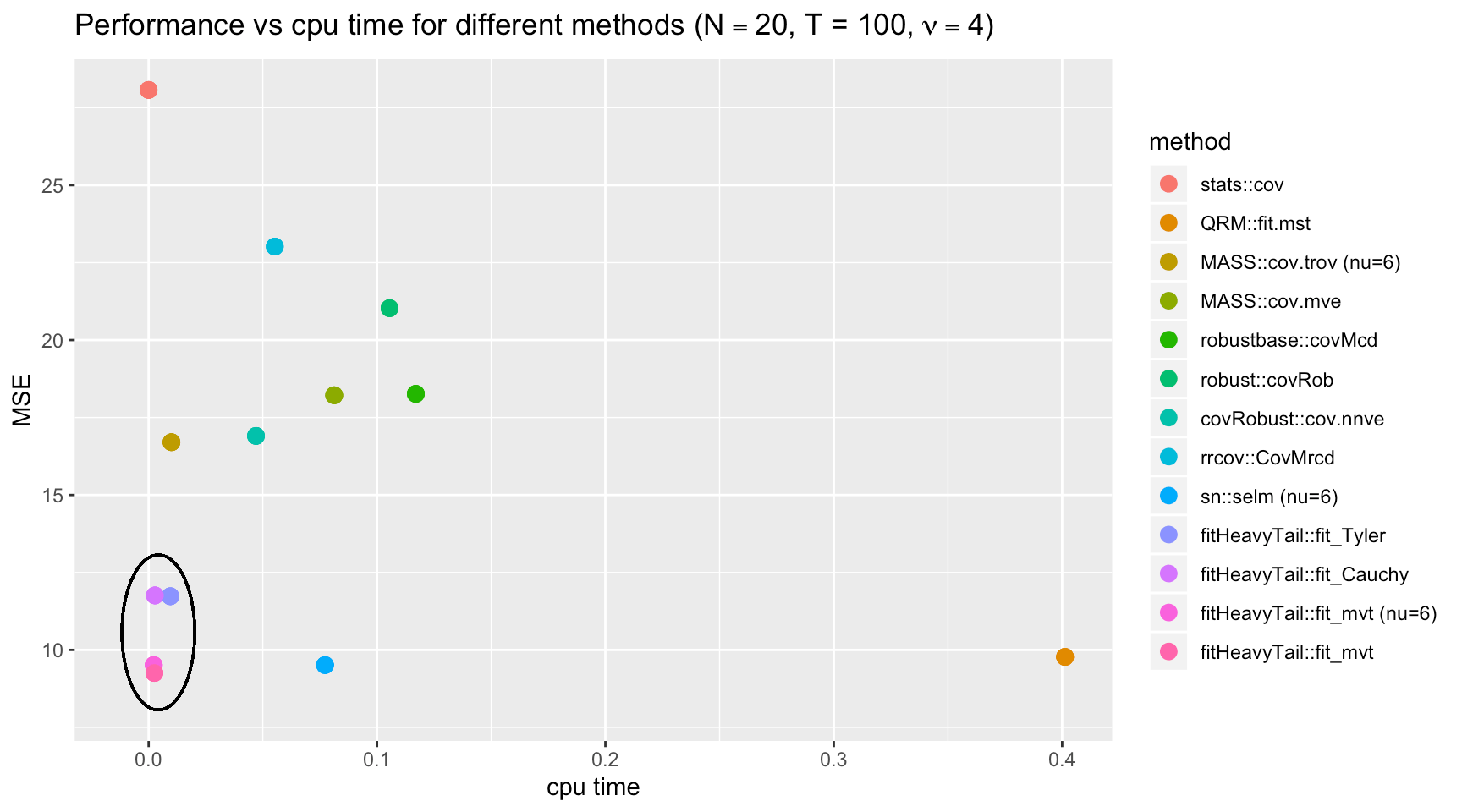# Mean Vector and Covariance Matrix Estimation under Heavy Tails

### 2019-12-31

This vignette illustrates the usage of the package fitHeavyTail to estimate the mean vector and covariance matrix of heavy-tailed multivariate distributions such as the angular Gaussian, Cauchy, or Student’s $$t$$ distribution. The results are compared against existing benchmark functions from different packages.

# Installation

The package can be installed from CRAN or GitHub:

# install stable version from CRAN
install.packages("fitHeavyTail")

# install development version from GitHub
devtools::install_github("dppalomar/fitHeavyTail")

# Getting help
library(fitHeavyTail)
help(package = "fitHeavyTail")
?fit_mvt

# Quick Start

To illustrate the simple usage of the package fitHeavyTail, let’s start by generating some multivariate data under a Student’s $$t$$ distribution with significant heavy tails ($$\nu=4$$):

library(mvtnorm)  # package for multivariate t distribution
N <- 10   # number of variables
T <- 80   # number of observations
nu <- 4   # degrees of freedom for tail heavyness

set.seed(42)
mu <- rep(0, N)
U <- t(rmvnorm(n = round(0.3*N), sigma = 0.1*diag(N)))
Sigma_cov <- U %*% t(U) + diag(N)  # covariance matrix with factor model structure
Sigma_scatter <- (nu-2)/nu * Sigma_cov
X <- rmvt(n = T, delta = mu, sigma = Sigma_scatter, df = nu)  # generate data

We can first estimate the mean vector and covariance matrix via the traditional sample estimates (i.e., sample mean and sample covariance matrix):

mu_sm     <- colMeans(X)
Sigma_scm <- cov(X)

Then we can compute the robust estimates via the package fitHeavyTail:

library(fitHeavyTail)
fitted <- fit_mvt(X)

We can now compute the estimation errors and see the big improvement:

sum((mu_sm     - mu)^2)
#>  0.2857323
sum((fitted$mu - mu)^2) #>  0.1404855 sum((Sigma_scm - Sigma_cov)^2) #>  5.861138 sum((fitted$cov - Sigma_cov)^2)
#>  4.107825

To get a visual idea of the robustness, we can plot the shapes of the covariance matrices (true and estimated ones) projected on two dimensions. Observe how the heavy-tailed estimation follows the true one more closely than the sample covariance matrix:# Numerical Comparison with Existing Packages

In the following, we generate multivariate heavy-tailed Student’s $$t$$ distributed data and compare the performance of many different existing packages via 100 Monte Carlo simulations in terms of estimation accurary, measured by the mean squared error (MSE), and cpu time.The following plot gives a nice overall perspective of the MSE vs. cpu time tradeoff of the different methods (note the ellipse at the bottom left that embraces the best four methods: fitHeavyTail::fit_Tyler(), fitHeavyTail::fit_Cauchy(), fitHeavyTail::fit_mvt(), and fitHeavyTail::fit_mvt() with fixed nu = 6):From the numerical results we can draw several observations:

• stats:cov() is the sample covariance matrix (SCM). As expected, it is not robust to heavy tails and has the worst estimation error although it enjoys the lowest computational cost. It is not acceptable for heavy-tailed distributions.
• QRM::fit.mst() assumes the data follows a multivariate $$t$$ distribution; it has one of the highest computational cost with a not-so-good estimation error.
• MASS::cov.trob() (with fixed nu = 6) assumes the data follows a multivariate $$t$$ distribution; it shows a good performance in terms of MSE and cpu time. It is probably the best choice among the benchmark existing packages (with the advantage that it comes preinstalled with base R in the package MASS).
• MASS::cov.mve() shows one of the worst performance in terms of both estimation error and computational cost.
• robustbase::covMcd() also shows one of the worst performance in terms of both estimation error and computational cost.
• robust::covRob() has a low computational cost but bad estimation error.
• covRobust::cov.nnve() shows a bad performance in terms of both estimatior error and cpu time.
• rrcov::CovMrcd() also shows one of the worst performance in terms of both estimation error and computational cost.
• sn::selm (nu=6) has a very good performance but with a high computational cost.
• fitHeavyTail::fit_Tyler() normalizes the data (to get rid of the shape of the tail); it shows a very small estimation error with an acceptable computational cost.
• fitHeavyTail::fit_Cauchy() assumes a multivariate Cauchy distribution and it has a performance similar to fitHeavyTail::fit_Tyler() but with a slightly higher computational cost.
• fitHeavyTail::fit_mvt() assumes the data follows a multivariate $$t$$ distribution; it shows a small estimation error with acceptable computational cost.
• fitHeavyTail::fit_mvt() with fixed nu = 6 seems to perform similar to the previous case (which also estimates nu).

Concluding, the top choices seem to be (in order):

1. fitHeavyTail::fit_mvt() (either without fixing nu or with nu = 6),
2. fitHeavyTail::fit_Cauchy(),
3. fitHeavyTail::fit_Tyler(), and
4. MASS::cov.trob() (with the advantage of being preinstalled with base R, but with a worse estimation error).

The overall winner is fitHeavyTail::fit_mvt() by a big margin.

# Algorithms

In essence, all the algorithms are based on the maximum likelihood estimation (MLE) of some assumed distribution given the observed data. The difficulty comes from the fact that the optimal solution to such MLE formulations becomes too involved in the form of a fixed-point equation and the framework of Majorization-Minimization (MM) algorithms  becomes key to derive efficient algorithms.

In some cases, the probability distribution function becomes too complicated to manage directly (like the multivariate Student’s $$t$$ distribution) and it is necessary to resort to a hierarchical distribution that involves some latent variables. In order to deal with such hidden variables, one has to resort to the Expectation-Maximization (EM) algorithm, which interestingly is an instance of the MM algorithm.

The following is a description of the algorithms used by the three fitting functions (note that the current version of the R package fitHeavyTail does not allow yet a regularization term with a target):

• The function fitHeavyTail::fit_Tyler() normalizes the centered samples $$\bar{\mathbf{x}}_t = \mathbf{x}_t - \boldsymbol{\mu}$$ (where $$\boldsymbol{\mu}$$ has been previously estimated), which then have an angular Gaussian distribution on the sphere, and performs an MLE based on the MM algorithm . The formulation including a regularization term is \begin{array}{ll} \underset{\boldsymbol{\Sigma}}{\textsf{minimize}} & \begin{aligned}[t] \frac{T}{2}\log\det(\boldsymbol{\Sigma}) + \frac{N}{2}\sum\limits_{t=1}^{T}\log{\left(\bar{\mathbf{x}}_t^T\boldsymbol{\Sigma}^{-1}\bar{\mathbf{x}}_t\right)}\hspace{2cm}\\ \color{darkred}{+ \;\alpha \left(N\log\left(\textsf{Tr}\left(\boldsymbol{\Sigma}^{-1}\mathbf{T}\right)\right) + \log\det(\boldsymbol{\Sigma})\right)} \end{aligned} \end{array} where $$\mathbf{T}$$ is the target matrix (e.g., $$\mathbf{T} = \mathbf{I}$$ or $$\mathbf{T} = \frac{1}{N}\textsf{Tr}(\mathbf{S})\times\mathbf{I}$$, with $$\mathbf{S}$$ being the sample covariance matrix). This leads to the iteration step $\boldsymbol{\Sigma}_{k+1} = (1 - \rho)\frac{N}{T}\sum\limits_{t=1}^{T}\frac{\bar{\mathbf{x}}_t\bar{\mathbf{x}}_t^T}{\bar{\mathbf{x}}_t^T\boldsymbol{\Sigma}_k^{-1}\bar{\mathbf{x}}_t} + \rho\frac{N}{\textsf{Tr}\left(\boldsymbol{\Sigma}_k^{-1}\mathbf{T}\right)}\mathbf{T},$ where $$\rho = \frac{\alpha}{T/2 + \alpha}$$ or $$\alpha = \frac{T}{2}\frac{\rho}{1 - \rho}$$, and initial point $$\boldsymbol{\Sigma}_{0} = \mathbf{S}$$. For better numerical stability, one can further normalize the estimate at each iteration: $$\boldsymbol{\Sigma}_{k+1} \leftarrow \boldsymbol{\Sigma}_{k+1}/\textsf{Tr}\left(\boldsymbol{\Sigma}_{k+1}\right)$$. The iterations converge to the solution up to a scaling factor if and only if $$1 + \frac{2}{T}\alpha > \frac{N}{T}$$ or, equivalently, $$\rho > 1 - \frac{T}{N}$$  (the correct scaling factor is later obtained via a robust fitting method). If instead the regularization term $$\color{darkred}{\textsf{Tr}\left(\boldsymbol{\Sigma}^{-1}\mathbf{T}\right) + \log\det(\boldsymbol{\Sigma})}$$ is used, the iteration step becomes $\boldsymbol{\Sigma}_{k+1} = (1 - \rho)\frac{N}{T}\sum\limits_{t=1}^{T}\frac{\bar{\mathbf{x}}_t\bar{\mathbf{x}}_t^T}{\bar{\mathbf{x}}_t^T\boldsymbol{\Sigma}_k^{-1}\bar{\mathbf{x}}_t} + \rho\mathbf{T}.$

• The function fitHeavyTail::fit_Cauchy() assumes that the data follows a multivariate Cauchy distribution ($$t$$ distribution with $$\nu=1$$) and performs an MLE based on the MM algorithm . The formulation including a regularization term is \begin{array}{ll} \underset{\boldsymbol{\mu},\boldsymbol{\Sigma}}{\textsf{minimize}} & \begin{aligned}[t] & \frac{T}{2}\log\det(\boldsymbol{\Sigma}) + \frac{N+1}{2}\sum\limits_{t=1}^{T}\log{\left(1+(\mathbf{x}_t - \boldsymbol{\mu})^T\boldsymbol{\Sigma}^{-1}(\mathbf{x}_t - \boldsymbol{\mu})\right)}\\ & \color{darkred}{+\;\alpha \left(N\log\left(\textsf{Tr}\left(\boldsymbol{\Sigma}^{-1}\mathbf{T}\right)\right) + \log\det(\boldsymbol{\Sigma})\right) + \gamma \log{\left(1 + (\boldsymbol{\mu} - \mathbf{t})^T\boldsymbol{\Sigma}^{-1}(\boldsymbol{\mu} - \mathbf{t})\right)}} \end{aligned} \end{array} where $$\mathbf{t}$$ and $$\mathbf{T}$$ are the targets for $$\boldsymbol{\mu}$$ and $$\boldsymbol{\Sigma}$$, respectively. This leads to the following (accelerated) iteration step (Algorithm 4 in ): $\boldsymbol{\mu}_{k+1} = \frac{(N+1)\sum_{t=1}^Tw_t\left(\boldsymbol{\mu}_k,\boldsymbol{\Sigma}_k\right)\mathbf{x}_t + 2\gamma w_{\textsf{tgt}}\left(\boldsymbol{\mu}_k,\boldsymbol{\Sigma}_k\right)\mathbf{t}}{(N+1)\sum_{t=1}^Tw_t\left(\boldsymbol{\mu}_k,\boldsymbol{\Sigma}_k\right) + 2\gamma w_{\textsf{tgt}}\left(\boldsymbol{\mu}_k,\boldsymbol{\Sigma}_k\right)}$ and $\boldsymbol{\Sigma}_{k+1} = \beta_k \left\{ (1 - \rho)\frac{N+1}{T}\sum\limits_{t=1}^{T}w_t\left(\boldsymbol{\mu}_k,\boldsymbol{\Sigma}_k\right)\left(\mathbf{x}_t - \boldsymbol{\mu}_{k+1}\right)\left(\mathbf{x}_t - \boldsymbol{\mu}_{k+1}\right)^T\\\hspace{6cm} + \rho\left(\frac{N}{\textsf{Tr}\left(\boldsymbol{\Sigma}_k^{-1}\mathbf{T}\right)}\mathbf{T} + \frac{\gamma}{\alpha}w_\textsf{tgt}\left(\boldsymbol{\mu}_k,\boldsymbol{\Sigma}_k\right)\left(\mathbf{t} - \boldsymbol{\mu}_{k+1}\right)\left(\mathbf{t} - \boldsymbol{\mu}_{k+1}\right)^T\right) \right\}$ where $$\rho = \frac{\alpha}{T/2 + \alpha}$$, \begin{aligned} w_t\left(\boldsymbol{\mu},\boldsymbol{\Sigma}\right) &= \frac{1}{1 + \left(\mathbf{x}_t - \boldsymbol{\mu}\right)^T\boldsymbol{\Sigma}^{-1}\left(\mathbf{x}_t - \boldsymbol{\mu}\right)},\\ w_\textsf{tgt}\left(\boldsymbol{\mu},\boldsymbol{\Sigma}\right) &= \frac{1}{1 + \left(\mathbf{t} - \boldsymbol{\mu}\right)^T\boldsymbol{\Sigma}^{-1}\left(\mathbf{t} - \boldsymbol{\mu}\right)},\\ \beta_k &= \frac{T+2\gamma}{(N+1)\sum_{t=1}^{T}w_t\left(\boldsymbol{\mu}_k,\boldsymbol{\Sigma}_k\right) + 2\gamma w_\textsf{tgt}\left(\boldsymbol{\mu}_k,\boldsymbol{\Sigma}_k\right)}, \end{aligned} and initial point $$\boldsymbol{\mu}_{0} = \frac{1}{T}\sum_{t=1}^{T}\mathbf{x}_t$$ and $$\boldsymbol{\Sigma}_{0} = \mathbf{S}$$ (note that this initial point is not totally correct due to a scaling factor). The iterations converge to the solution if and only if the conditions of Corollary 3 in  are satisfied.

• The function fitHeavyTail::fit_mvt() assumes the data follows a multivariate Student’s $$t$$ distribution and performs an MLE based on the EM algorithm . The MLE formulation (without missing values) is \begin{array}{ll} \underset{\boldsymbol{\mu},\boldsymbol{\Sigma},\nu}{\textsf{minimize}} & \begin{aligned}[t] \frac{T}{2}\log\det(\boldsymbol{\Sigma}) + \frac{N+\nu}{2}\sum\limits_{t=1}^{T}\log{\left(1+\frac{1}{\nu}(\mathbf{x}_t - \boldsymbol{\mu})^T\boldsymbol{\Sigma}^{-1}(\mathbf{x}_t - \boldsymbol{\mu})\right)}\\ -\; T\log{\Gamma\left(\frac{N+\nu}{2}\right)} + T\log{\Gamma\left(\frac{\nu}{2}\right)} + \frac{TN}{2}\log{\nu}. \end{aligned} \end{array} Since its direct minimization is complicated, the EM algorithm instead iteratively optimizes the Q function at iteration $$k$$: \begin{array}{ll} \underset{\boldsymbol{\mu},\boldsymbol{\Sigma},\nu}{\textsf{minimize}} & \begin{aligned}[t] \frac{T}{2}\log\det(\boldsymbol{\Sigma}) + \sum\limits_{t=1}^{T}\left\{\frac{\textsf{E}_k[\tau_t]}{2}(\mathbf{x}_t - \boldsymbol{\mu})^T\boldsymbol{\Sigma}^{-1}(\mathbf{x}_t - \boldsymbol{\mu}) + \frac{\nu}{2}\textsf{E}_k[\tau_t] - \frac{\nu}{2}\textsf{E}_k[\log{\tau_t]}\right\}\\ -\; \frac{T\nu}{2}\log{\frac{\nu}{2}} + T\log{\Gamma\left(\frac{\nu}{2}\right)} \end{aligned} \end{array} where $\textsf{E}_k[\tau_t] = \frac{\nu_k + N}{\nu_k + \left(\mathbf{x}_t - \boldsymbol{\mu}_k\right)^T\boldsymbol{\Sigma}_k^{-1}\left(\mathbf{x}_t - \boldsymbol{\mu}_k\right)}.$ The (accelerated) solution is given by $\boldsymbol{\mu}_{k+1} = \frac{\sum_{t=1}^T\textsf{E}_k[\tau_t]\mathbf{x}_t}{\sum_{t=1}^T\textsf{E}_k[\tau_t]},$ $\boldsymbol{\Sigma}_{k+1} = \frac{1}{\alpha_k}\frac{1}{T}\sum_{t=1}^{T}\textsf{E}_k[\tau_t]\left(\mathbf{x}_t - \boldsymbol{\mu}_{k+1}\right)\left(\mathbf{x}_t - \boldsymbol{\mu}_{k+1}\right)^T,$ with $$\alpha_k = \frac{1}{T}\sum_{t=1}^T\textsf{E}_k[\tau_t]$$, and $$\nu_{k+1}$$ can be found by a one-dimensional search:

• method ECM based on the Q function:

$\nu_{k+1} = \arg\min_\nu \left\{\frac{\nu}{2}\sum_{t=1}^{T}\left(\textsf{E}_k[\tau_t] - \textsf{E}_k[\log{\tau_t]}\right) - \frac{\nu}{2}T\log{\frac{\nu}{2}} + T\log{\Gamma\left(\frac{\nu}{2}\right)}\right\}$

• method ECME based directly on the likelihood:

$\nu_{k+1} = \arg\min_\nu \left\{ \frac{N + \nu}{2}\sum_{t=1}^{T}\log{\left(\nu + \left(\mathbf{x}_t - \boldsymbol{\mu}_{k+1}\right)\boldsymbol{\Sigma}_{k+1}^{-1}\left(\mathbf{x}_t - \boldsymbol{\mu}_{k+1}\right)^T\right)}\\\hspace{6cm} - T\log{\Gamma\left(\frac{N + \nu}{2}\right)} + T\log{\Gamma\left(\frac{\nu}{2}\right)} - \frac{\nu}{2}T\log{\nu} \right\}.$ The initial point is $$\boldsymbol{\mu}_{0} = \frac{1}{T}\sum_{t=1}^{T}\mathbf{x}_t$$, $$\boldsymbol{\Sigma}_{0} = \frac{\nu_0-2}{\nu_0}\mathbf{S}$$, and $$\nu_0 = 2/\kappa + 4$$, with $$\kappa = \left[\frac{1}{3}\frac{1}{N}\sum_{i=1}^N \textsf{kurt}_i\right]^+$$ and $\textsf{kurt}_i = \frac{(T-1)}{(T-2)(T-3)}\left((T+1)\left(\frac{m_i^{(4)}}{\big(m_i^{(2)}\big)^2} - 3\right) + 6\right),$ where $$m_i^{(q)}=\frac{1}{T}\sum_{t=1}^T(x_{it}-\bar{x}_i)^q$$ denotes the $$q$$th order sample moment. The algorithm with missing values in $$\mathbf{x}_t$$ becomes more cumbersome but it is essentially the same idea. This function can also incorporate a factor model structure on the covariance matrix $$\boldsymbol{\Sigma} = \mathbf{B}\mathbf{B}^T + {\sf Diag}(\boldsymbol{\psi})$$, which requires a more sophisticated algorithm  (available in arXiv).